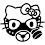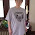## Thursday, July 14, 2016

### Magic Square 26 x 26.. Sum of any square 8801

God x God

Notice 666 is 23 down 10 across... 23+10=33 interesting...

1.This is interesting. I've thrown it in the spreadsheet. I'll have to meditate on it a little to see how I can apply it.
Thank you for sharing!

1.This comment has been removed by the author.

2.Sorry. Forgot tabs don't work so well here in comments...
4,17=11
4+17=21
4*17=68
1,21=22
1+21=22
1*21=21
11,12=33
11+12=23
11*12=132
8,3=44
8+3=11
8*3=24
5,20=55
5+20=25
5*20=100
2,24=66
2+24=26
2*24=48
13,13=77
13+13=26
13*13=169
10,4=88
10+4=14
10*4=40
7,8=99
7+8=15
7*8=56
5,24=111
5+24=11
5*24=120
22,16=222
22+15=38
22*15=330
18,15=333
18+15=33
18*15=270
16,26=444
16+26=42
16*26=416
25,12=555
25+12=37
25*12=300
10,23=666
10+23=33
10*23=230

21,17=223
21+17=38
21*17=357
18,14=322
18+14=32
18*14=252
15,23=234
15+23=38
15*23=345
15,13=432
15+13=28
15*13=195

3.18 15=333 Remember Prince Harry cellphone time in the Invictus video? 18:15
18,15=333
18+15=33
PRINCE HARRY & QUEEN ELIZABETH II GET LAST LAUGH in Invictus Games Rivalry with Obama's

4.No, I totally didn't remember!
Damn, between you and Zach, ya'll have the memories!
Thanks for reminding!

5.I should have asked... do you mind me adding it to my spreadsheet? It is the spreadsheet that I share out from my Google Drive for whoever wants it? I'd already put your name/blog URL in, but before I share it, are you okay with that?

6.2.Is there something special about 541?

3.541 Jewish
Fibonacci Sequence, Prime number, pray

1.4.